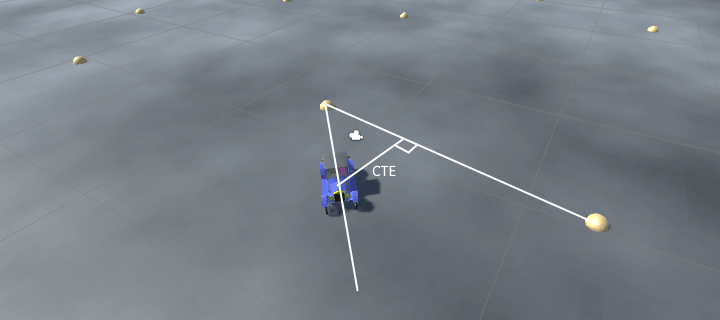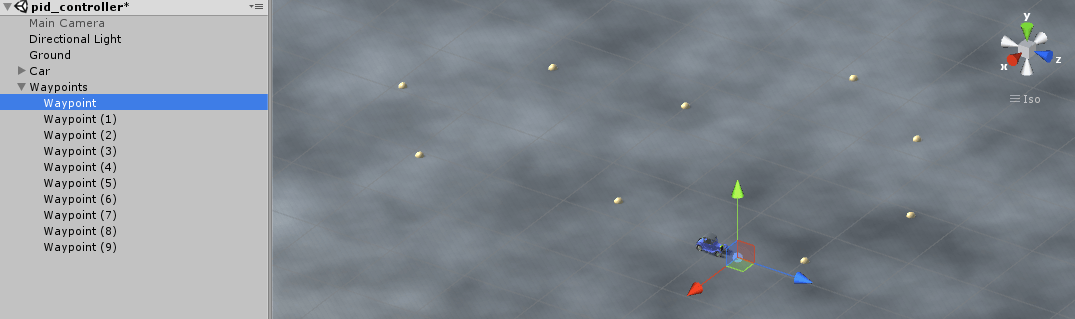# Minimize an error with a PID controller in Unity with C#

## 1. Improve the steering of a self-driving car

This is a tutorial on how you can minimize an error with the help of a PID controller. This is a technique used in real-life self-driving cars and I learned about it in the free course Artificial Intelligence for Robotics - Programming a Robotic Car.

The error we are going to minimize here is the error a self-driving car has when it's following a series of waypoints. This error is called Cross Track Error, or simply CTE, and is the distance between the car's actual position and the position it should have, which is the position closest to the car on a line between the waypoints:Why do you need to minimize this error? You could just determine if the car should steer left or right to reach the waypoint(as explained here: Turn left or right to reach a waypoint?). This is working, but the wheels will move really fast to the left or right when the car is driving straight towards the waypoint, which is not looking good. To minimize this behavior, a good way is to take the rolling average of the steering angles, but now the car will look like it's drunk. So we need a better way, which is a PID controller.

### Code

First of all you need a car, and you can use Unity's wheelcollider example WheelCollider Tutorial. Then you need a series of waypoints. Everything should look like this:The first script you have to attach to the car. To this script you have to add all waypoints (in the correct order), and the wheels.

```using UnityEngine;
using System.Collections;
using System.Collections.Generic;

//Modified basic car controller from Unity
//https://docs.unity3d.com/Manual/WheelColliderTutorial.html

[System.Serializable]
public class AxleInfo
{
public WheelCollider leftWheel;
public WheelCollider rightWheel;
public bool motor;
public bool steering;
}

public class CarController : MonoBehaviour
{
public List axleInfos;

//Car data
float maxMotorTorque = 500f;
float maxSteeringAngle = 40f;

//To get a more realistic behavior
public Vector3 centerOfMassChange;

//The difference between the center of the car and the position where we steer
public float centerSteerDifference;
//The position where the car is steering
private Vector3 steerPosition;

//All waypoints
public List allWaypoints;
//The current index of the list with all waypoints
private int currentWaypointIndex = 0;
//The waypoint we are going towards and the waypoint we are going from
private Vector3 currentWaypoint;
private Vector3 previousWaypoint;

//Average the steering angles to simulate the time it takes to turn the wheel
float averageSteeringAngle = 0f;

PIDController PIDControllerScript;

void Start()
{
//Move the center of mass
transform.GetComponent().centerOfMass = transform.GetComponent().centerOfMass + centerOfMassChange;

//Init the waypoints
currentWaypoint = allWaypoints[currentWaypointIndex].position;

previousWaypoint = GetPreviousWaypoint();

PIDControllerScript = GetComponent();
}

//Finds the corresponding visual wheel, correctly applies the transform
void ApplyLocalPositionToVisuals(WheelCollider collider)
{
if (collider.transform.childCount == 0)
{
return;
}

Transform visualWheel = collider.transform.GetChild(0);

Vector3 position;
Quaternion rotation;
collider.GetWorldPose(out position, out rotation);

visualWheel.transform.position = position;
visualWheel.transform.rotation = rotation;
}

void Update()
{
//So we can experiment with the position where the car is checking if it should steer left/right
//doesn't have to be where the wheels are - especially if we are reversing
steerPosition = transform.position + transform.forward * centerSteerDifference;

//Check if we should change waypoint
if (Math.HasPassedWaypoint(steerPosition, previousWaypoint, currentWaypoint))
{
currentWaypointIndex += 1;

if (currentWaypointIndex == allWaypoints.Count)
{
currentWaypointIndex = 0;
}

currentWaypoint = allWaypoints[currentWaypointIndex].position;

previousWaypoint = GetPreviousWaypoint();
}
}

//Get the waypoint before the current waypoint we are driving towards
Vector3 GetPreviousWaypoint()
{
previousWaypoint = Vector3.zero;

if (currentWaypointIndex - 1 < 0)
{
previousWaypoint = allWaypoints[allWaypoints.Count - 1].position;
}
else
{
previousWaypoint = allWaypoints[currentWaypointIndex - 1].position;
}

return previousWaypoint;
}

void FixedUpdate()
{
float motor = maxMotorTorque;

//Manual controls for debugging
//float motor = maxMotorTorque * Input.GetAxis("Vertical");
//float steering = maxSteeringAngle * Input.GetAxis("Horizontal");

//
//Calculate the steering angle
//
//The simple but less accurate way -> will produce drunk behavior
//float steeringAngle = maxSteeringAngle * Math.SteerDirection(transform, steerPosition, currentWaypoint);

//Get the cross track error, which is what we want to minimize with the pid controller
float CTE = Math.GetCrossTrackError(steerPosition, previousWaypoint, currentWaypoint);

//But we still need a direction to steer
CTE *= Math.SteerDirection(transform, steerPosition, currentWaypoint);

float steeringAngle = PIDControllerScript.GetSteerFactorFromPIDController(CTE);

//Limit the steering angle
steeringAngle = Mathf.Clamp(steeringAngle, -maxSteeringAngle, maxSteeringAngle);

//Average the steering angles to simulate the time it takes to turn the steering wheel
float averageAmount = 30f;

averageSteeringAngle = averageSteeringAngle + ((steeringAngle - averageSteeringAngle) / averageAmount);

//
//Apply everything to the car
//
foreach (AxleInfo axleInfo in axleInfos)
{
if (axleInfo.steering)
{
axleInfo.leftWheel.steerAngle = averageSteeringAngle;
axleInfo.rightWheel.steerAngle = averageSteeringAngle;
}
if (axleInfo.motor)
{
axleInfo.leftWheel.motorTorque = motor;
axleInfo.rightWheel.motorTorque = motor;
}

ApplyLocalPositionToVisuals(axleInfo.leftWheel);
ApplyLocalPositionToVisuals(axleInfo.rightWheel);
}
}
}

```

Next up is the PID controller, which you also have to attach to the car.

```using UnityEngine;
using System.Collections;

public class PIDController : MonoBehaviour
{
float CTE_old = 0f;
float CTE_sum = 0f;

//PID parameters
public float tau_P = 0f;
public float tau_I = 0f;
public float tau_D = 0f;

public float GetSteerFactorFromPIDController(float CTE)
{
//The steering factor
float alpha = 0f;

//P
alpha = tau_P * CTE;

//I
CTE_sum += Time.fixedDeltaTime * CTE;

//Sometimes better to just sum the last errors
float averageAmount = 20f;

CTE_sum = CTE_sum + ((CTE - CTE_sum) / averageAmount);

alpha += tau_I * CTE_sum;

//D
float d_dt_CTE = (CTE - CTE_old) / Time.fixedDeltaTime;

alpha += tau_D * d_dt_CTE;

CTE_old = CTE;

return alpha;
}
}
```

The last script is the funny math you need to make all this work.

```using UnityEngine;
using System.Collections;

public static class Math
{
//Have we passed a waypoint?
public static bool HasPassedWaypoint(Vector3 carPos, Vector3 goingFromPos, Vector3 goingToPos)
{
bool hasPassedWaypoint = false;

//The vector between the character and the waypoint we are going from
Vector3 a = carPos - goingFromPos;

//The vector between the waypoints
Vector3 b = goingToPos - goingFromPos;

//Vector projection from https://en.wikipedia.org/wiki/Vector_projection
//To know if we have passed the upcoming waypoint we need to find out how much of b is a1
//a1 = (a.b / |b|^2) * b
//a1 = progress * b -> progress = a1 / b -> progress = (a.b / |b|^2)
float progress = (a.x * b.x + a.y * b.y + a.z * b.z) / (b.x * b.x + b.y * b.y + b.z * b.z);

//If progress is above 1 we know we have passed the waypoint
if (progress > 1.0f)
{
hasPassedWaypoint = true;
}

return hasPassedWaypoint;
}

//Should we turn left or right to reach the next waypoint?
public static float SteerDirection(Transform carTrans, Vector3 steerPosition, Vector3 waypointPos)
{
//The right direction of the direction you are facing
Vector3 youDir = carTrans.right;

//The direction from you to the waypoint
Vector3 waypointDir = waypointPos - steerPosition;

//The dot product between the vectors
float dotProduct = Vector3.Dot(youDir, waypointDir);

//Now we can decide if we should turn left or right
float steerDirection = 0f;
if (dotProduct > 0f)
{
steerDirection = 1f;
}
else
{
steerDirection = -1f;
}

return steerDirection;
}

//Get the distance between where the car is and where it should be
public static float GetCrossTrackError(Vector3 carPos, Vector3 goingFromPos, Vector3 goingToPos)
{
//The first part is the same as when we check if we have passed a waypoint

//The vector between the character and the waypoint we are going from
Vector3 a = carPos - goingFromPos;

//The vector between the waypoints
Vector3 b = goingToPos - goingFromPos;

//Vector projection from https://en.wikipedia.org/wiki/Vector_projection
//To know if we have passed the upcoming waypoint we need to find out how much of b is a1
//a1 = (a.b / |b|^2) * b
//a1 = progress * b -> progress = a1 / b -> progress = (a.b / |b|^2)
float progress = (a.x * b.x + a.y * b.y + a.z * b.z) / (b.x * b.x + b.y * b.y + b.z * b.z);

//The coordinate of the position where the car should be
Vector3 errorPos = goingFromPos + progress * b;

//The error between the position where the car should be and where it is
float error = (errorPos - carPos).magnitude;

return error;
}
}
```

And that's it, your passengers in your self-driving car don't need to get seasick anymore!